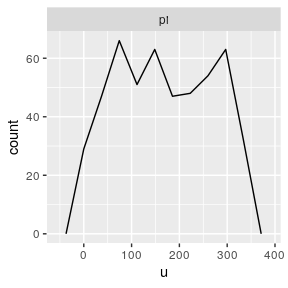# Simulation Based Calibration

#### 2023-01-16

Here is a Stan program for a beta-binomial model

data {
int<lower = 1> N;
real<lower = 0> a;
real<lower = 0> b;
}
transformed data { // these adhere to the conventions above
real pi_ = beta_rng(a, b);
int y = binomial_rng(N, pi_);
}
parameters {
real<lower = 0, upper = 1> pi;
}
model {
target += beta_lpdf(pi | a, b);
target += binomial_lpmf(y | N, pi);
}
generated quantities { // these adhere to the conventions above
int y_ = y;
vector pars_;
int ranks_ = {pi > pi_};
vector[N] log_lik;
pars_ = pi_;
for (n in 1:y) log_lik[n] = bernoulli_lpmf(1 | pi);
for (n in (y + 1):N) log_lik[n] = bernoulli_lpmf(0 | pi);
}

Notice that it adheres to the following conventions:

• Realizations of the unknown parameters are drawn in the transformed data block are postfixed with an underscore, such as pi_. These are considered the “true” parameters being estimated by the corresponding symbol declared in the parameters block, which have the same names except for the trailing underscore, such as pi.
• The realizations of the unknown parameters are then conditioned on when drawing from the prior predictive distribution in transformed data block, which in this case is int y = binomial_rng(N, pi_);. To avoid confusion, y does not have a training underscore.
• The realizations of the unknown parameters are copied into a vector in the generated quantities block named pars_
• The realizations from the prior predictive distribution are copied into an object (of the same type) in the generated quantities block named y_. This is optional.
• The generated quantities block contains an integer array named ranks_ whose only values are zero or one, depending on whether the realization of a parameter from the posterior distribution exceeds the corresponding “true” realization, which in this case is ranks_ = {pi > pi_};. These are not actually “ranks” but can be used afterwards to reconstruct (thinned) ranks.
• The generated quantities block contains a vector named log_lik whose values are the contribution to the log-likelihood by each observation. In this case, the “observations” are the implicit successes and failures that are aggregated into a binomial likelihood. This is optional but facilitates calculating the Pareto k shape parameter estimates that indicate whether the posterior distribution is sensitive to particular observations.

Assuming the above is compile to a code stanmodel named beta_binomial, we can then call the sbc function

output <- sbc(beta_binomial, data = list(N = 10, a = 1, b = 1), M = 500, refresh = 0)

At which point, we can then call

print(output)
## 0 chains had divergent transitions after warmup
plot(output, bins = 10) # it is best to specify the bins argument yourself`